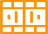## 一份用Python处理音频的实用指南：什么是梅尔频谱图，以及如何用通俗易懂的语言生成音视频。

1.最先进的技术-本文（声音及其数字化方式。在日常生活中深度学习音频会遇到那些问题？什么是声谱图，为什么它们对使用语音聊天室至关重要？）

2.为什么梅尔频谱图的性能更好（在Python中处理音频数据。什么是梅尔频谱图以及如何生成它们）

3.功能的优化和增强（增强频谱图功能可通过超参数调整和数据增强实现最佳性能）

4.音频分类（端到端的示例和用于对普通声音进行分类的体系结构。适用于语音聊天室使用的各种场景。）

5.自动语音识别（语音转文本算法和体系结构，使用CTC Loss和解码来对齐序列）

## 音频文件格式和Python库

Python中有一些很棒的音频处理库，其中，Librosa是最受欢迎的数据库之一，并且功能广泛，scipy也很常用。如果你使用的是Pytorch，它有一个名为torchaudio的配套库，该库与Pytorch紧密联系，它虽然没有Librosa那么的功能，但它却是专门为深度学习而开发的。

``````import librosa

AUDIO_FILE = './audio.wav'
``````

``````import librosa.display
import matplotlib.pyplot as plt

# x-axis has been converted to time using our sample rate.
# matplotlib plt.plot(y), would output the same figure, but with sample
# number on the x-axis instead of seconds
plt.figure(figsize=(14, 5))
librosa.display.waveplot(samples, sr=sample_rate)
``````

``````from IPython.display import Audio
Audio(AUDIO_FILE)
``````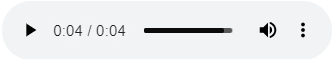## 音频信号数据

``````print ('Example shape ', samples.shape, 'Sample rate ', sample_rate, 'Data type', type(samples))
print (samples[22400:22420])
``````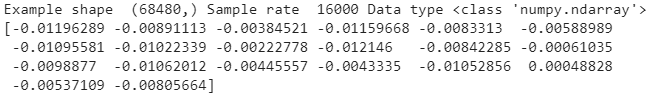## 频谱图

``````sgram = librosa.stft(samples)
librosa.display.specshow(sgram)

``````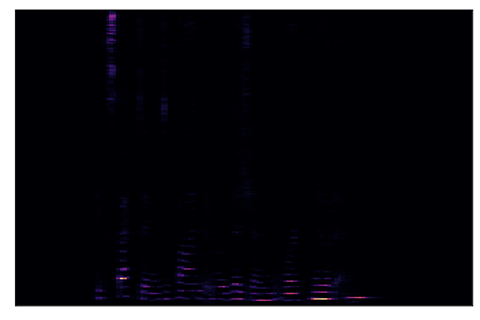## 人类如何听到频率？

• 100Hz和200Hz
• 1000Hz和1100Hz
• 10000Hz和10100 Hz

## 梅尔倒频谱系数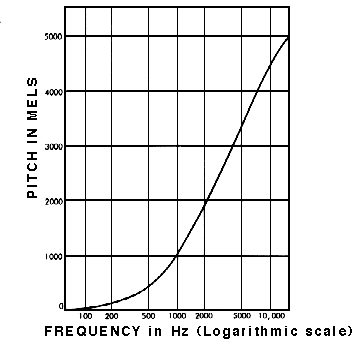## 分贝标度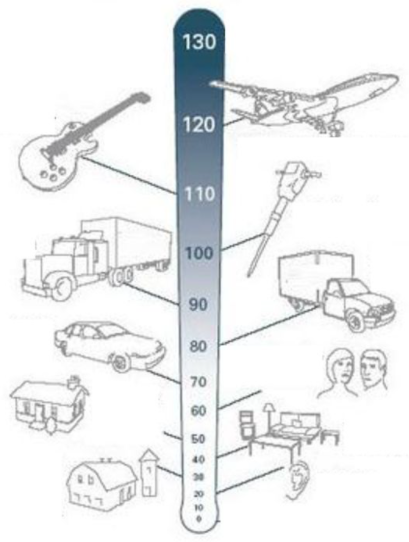## 梅尔谱图

• 它使用Mel比例而不是y轴上的频率。
• 它使用分贝标度代替振幅来指示颜色。

``````# use the mel-scale instead of raw frequency
sgram_mag, _ = librosa.magphase(sgram)
mel_scale_sgram = librosa.feature.melspectrogram(S=sgram_mag, sr=sample_rate)
librosa.display.specshow(mel_scale_sgram)
``````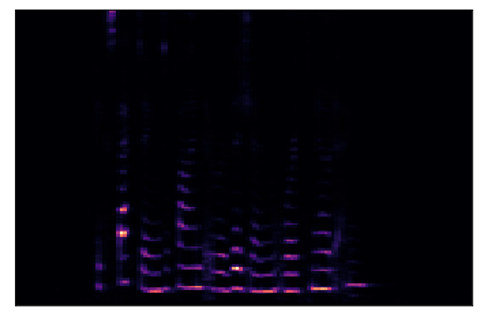``````# use the decibel scale to get the final Mel Spectrogram
mel_sgram = librosa.amplitude_to_db(mel_scale_sgram, ref=np.min)
librosa.display.specshow(mel_sgram, sr=sample_rate, x_axis='time', y_axis='mel')
plt.colorbar(format='%+2.0f dB')
``````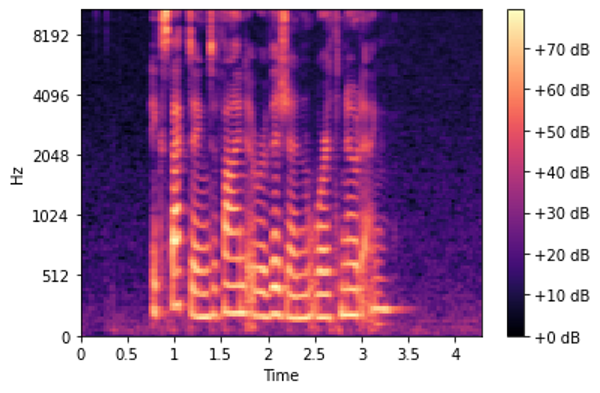## 结论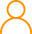AgoraTechnicalTeam
TA 暂未填写个人简介

131